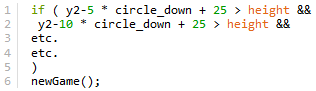#### Howdy, Stranger!

We are about to switch to a new forum software. Until then we have removed the registration on this forum.

# Restart when hitting the ellipses

edited November 2014

Hey, I'm taking a class in Processing, and we're making simple video games as one of our first projects. Mine is a square that is controlled with "a" and "d" to dodge the falling ellipses. I'm trying to make it so that 1. once all the ellipses reach the bottom, "circle_down" resets and they fall from the top again using the new variable, and 2. once the square touches an ellipse, the game stops. (Clearly, I don't have any idea how to make each line of code on its own line on the forum, so sorry about that...)

/* Move the square left and right with "a" and "d" to dodge the falling red ellipses. */

int x = 10;
int square_move = 30;
float circle_down = random (15, 25);
float x2 = random (10, 390);
float x3 = random (10, 390);
float x4 = random (10, 390);
float x5 = random (10, 390);
float x6 = random (10, 390);
float y2 = 20;

void setup() {
size(400, 400);
stroke(255);
frameRate (20);
}

void draw () {
background(255);
fill (0);
rect (x, 350, 20, 20);
circle ();
}

void keyPressed () { if (key == 'd') {
x = x + square_move;
} if (key == 'a') {
x = x - square_move;
}
}

void circle () {
fill (255,0,0);
y2 = y2 + circle_down;
ellipse (x2, y2-5 * circle_down, 25, 25);
ellipse (x3, y2-10 * circle_down, 25, 25);
ellipse (x4, y2-25 * circle_down, 25, 25);
ellipse (x5, y2-28 * circle_down, 25, 25);
ellipse (x6, y2-27 * circle_down, 25, 25);
ellipse (x2, y2- circle_down+2, 25, 25);
ellipse (x3+11, y2-32 * circle_down+5, 25, 25);
ellipse (x4+5, y2-15 * circle_down+8, 25, 25);
ellipse (x5+6, y2-29 * circle_down+9, 25, 25);
ellipse (x6+9, y2-31 * circle_down+6, 25, 25);
}

Thanks for your help! And, since this is obviously one of the clunkiest codes ever written, I'd appreciate any other suggestions as well.

Tagged:

• regarding formatting code here in the forum, read

http://forum.processing.org/two/discussion/8045/how-to-format-code-and-text#Item_1

here

``````/* Move the square left and right with "a" and "d" to dodge the falling red ellipses. */

int x = 10;
int square_move = 30;
float circle_down = random (15, 25);
float x2 = random (10, 390);
float x3 = random (10, 390);
float x4 = random (10, 390);
float x5 = random (10, 390);
float x6 = random (10, 390);
float y2 = 20;

void setup() {
size(400, 400);
stroke(255);
frameRate (20);
}

void draw () {
background(255);
fill (0);
rect (x, 350, 20, 20);
circle ();
}

void keyPressed () { if (key == 'd') {
x = x + square_move;
} if (key == 'a') {
x = x - square_move;
}
}

void circle () {
fill (255,0,0);
y2 = y2 + circle_down;
ellipse (x2, y2-5 * circle_down, 25, 25);
ellipse (x3, y2-10 * circle_down, 25, 25);
ellipse (x4, y2-25 * circle_down, 25, 25);
ellipse (x5, y2-28 * circle_down, 25, 25);
ellipse (x6, y2-27 * circle_down, 25, 25);
ellipse (x2, y2- circle_down+2, 25, 25);
ellipse (x3+11, y2-32 * circle_down+5, 25, 25);
ellipse (x4+5, y2-15 * circle_down+8, 25, 25);
ellipse (x5+6, y2-29 * circle_down+9, 25, 25);
ellipse (x6+9, y2-31 * circle_down+6, 25, 25);
}
``````

at the moment your code lacks flexibility since your ellipses have all their y-values written in plain text - it'd be easier to have them in arrays - see article about this ny PhiLho

http://forum.processing.org/two/discussion/8082/from-several-variables-to-arrays

1. once all the ellipses reach the bottom, "circle_down" resets and they fall from the top again using the new variable

yeah...

first check

``````if ( y2-5 * circle_down + 25 > height &&
y2-10 * circle_down + 25 > height &&
etc.
etc.
)
newGame();
``````

newGame is a function you have to write

it contains

``````x2 = random (10, 390);
x3 = random (10, 390);
x4 = random (10, 390);
x5 = random (10, 390);
x6 = random (10, 390);
y2 = 20;
``````
1. once the square touches an ellipse, the game stops.

err....

before setup() say boolean GameStopped = false;

once the square touches an ellipse say GameStopped = true;

in draw() say

``````if (GameStopped == false)
circle();
``````

once the square touches an ellipse

again, easier with arrays, read article

``````if ( dist (x2, y2-5 * circle_down, x,350) < 30 ||
dist (x2, y2-10 * circle_down, x,350) < 30 ||
etc etc
GameStopped = true;
``````

;-)

• .

• I'm sorry, I just started learning all this a about a month ago. I'm not really sure what you want foror where I would put it.